﻿ 机场出租车的司机决策与管理优化研究 Study on Driver Decision and Management Optimization of Airport Taxi

Statistics and Application
Vol. 09  No. 01 ( 2020 ), Article ID: 33742 , 12 pages
10.12677/SA.2020.91002

Study on Driver Decision and Management Optimization of Airport Taxi

Ruiqian Li, Yufei Cao, Zhipeng Liu

Xidian University, Xi’an Shaanxi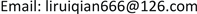Received: Dec. 11th, 2019; accepted: Dec. 26th, 2019; published: Jan. 2nd, 2020ABSTRACT

In order to realize the scientific dispatching of airport taxis and balance the revenue of long-distance and short-distance taxis. According to the daily data released by the monitoring and operation center of CAAC, this paper establishes a prediction model of the passenger volume in the taxi storage pool of the airport. Furthermore, based on the psychology of taxi drivers, the paper establishes a deterministic decision-making model of whether drivers choose to wait or not. Concerning about the analysis of the dependence of the decision model, we find that the decision-making of night drivers is more dependent on the number of flights in the near period, while that of day drivers is more dependent on the number of taxis queuing in the car storage pool. Based on the objective programming model, a priority arrangement scheme for airport short-distance drivers is proposed. This scheme will improve the current situation of income difference between long and short-distance passengers and help short-distance passengers avoid the risk of being “rejected” and being treated unfairly.

Keywords:Deterministic Decision, Expected Revenue, Dependency Analysis, Goal Programming, Priority Right1. 引言

1) 分析研究与出租车司机决策相关因素的影响机理，综合考虑机场乘客数量的变化规律和出租车司机的收益，建立出租车司机选择决策模型，并给出司机的选择策略。

2) 机场的出租车载客收益与载客的行驶里程有关，乘客的目的地有远有近，出租车司机不能选择乘客和拒载，但允许出租车多次往返载客。如果给对某些短途载客再次返回的出租车给予一定的“优先权”，可以使得出租车的收益尽量均衡。本文试给出一个可行的“优先权”安排方案。

2. 问题的剖析与思路建立

2.1. 问题一的分析

2.2. 问题二的分析

2.3. 模型的假设

1) 假设出租车司机均为理性人，在知道不同方案预期收益时，会选择收益更大的方案。

2) 各出租车间的载人盈利情况相互独立，互不影响。

3) 忽略司机在蓄车池排队时低速移动的油耗费用。

4) 在某时间段抵达的航班数量和“蓄车池”里已有的车辆数是司机可观测到的确定信息。

2.4. 符号说明

3. 出租车司机的决策方案

3.1. 决策系统与选择策略

$\left\{\begin{array}{l}{a}_{1}:等待,\text{\hspace{0.17em}}\text{\hspace{0.17em}}即前往蓄车池排队等候\\ {a}_{2}:不等,\text{\hspace{0.17em}}\text{\hspace{0.17em}}即从机场直接回到市区\end{array}$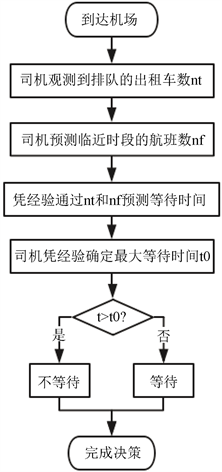Figure 1. Driver decision flow chart

3.2. 决策机理的分析

3.2.1. 等待时间的预测Figure 2. Chart of number of passengers and number of flights with time

$V\left(t\right)=\alpha {n}_{f}\left(t\right)$(1)

t时刻出站的乘客中选择乘坐出租车前往市区的比例为β，可以得到前往乘车区的乘客数为βαnf (t)。这部分人到达乘车区的时间分布为偏态分布，那么可以得到乘车区的每分钟客流量np (t)。假设乘车区的车数为nw，每一批次乘车区的出租车运载的乘客数为λnw，λ表示出租车的平均载客量，这与乘客出行的结伴情况有关。机场出租车管理人员对出租车实行“分批定量”放行进入“乘车区”。那么需要等待的批次数为：

$k=⌊\frac{{n}_{t}}{{n}_{w}}⌋+\text{1}$(2)

$\underset{i=1}{\overset{n}{\sum }}{n}_{p}\left({t}_{i}\right)=\lambda k{n}_{w}$(3)

3.2.2. 最大等待时间的给出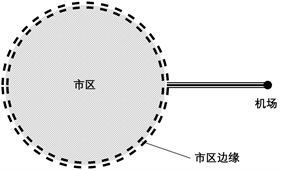(a)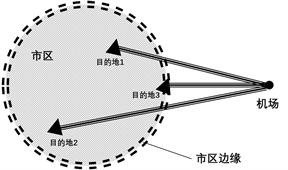(b)

Figure 3. Spatial relationship between airport and urban area

$E\left({w}_{a2}\right)=-{v}_{a}{t}_{a}{r}_{o}$(4)

$E\left({w}_{c\text{2}}\right)=\left(\text{1}-p\right){r}_{i}{t}_{c\text{2}}{v}_{c}-p{r}_{o}{t}_{c\text{2}}{v}_{c}$(5)

$\begin{array}{c}E\left({w}_{2}\right)=E\left({w}_{a2}\right)+E\left({w}_{c2}\right)\\ ={r}_{i}\left[\left(1-p\right){t}_{c\text{2}}{v}_{c}\right]-{r}_{o}\left[p{t}_{c\text{2}}{v}_{c}+{t}_{a}{v}_{a}\right]\end{array}$(6)

$E\left({w}_{a1}\right)+E\left({w}_{c1}\right)={r}_{i}\left({t}_{a}{v}_{a}+{t}_{c1}{v}_{c}\right)$(7)

$E\left({w}_{1}\right)={r}_{i}\left({t}_{a}{v}_{a}+{t}_{c1}{v}_{c}\right)$ (8)

$E\left({w}_{1}\right)={r}_{i}\left({s}_{\text{1}}+{s}_{2}\right)$ (9)

$\begin{array}{c}E\left({w}_{2}\right)={r}_{i}\left[\left(1-p\right)\left({t}_{b\text{1}}+{t}_{c1}\right){v}_{c}\right]-{r}_{o}\left[p\left({t}_{b\text{1}}+{t}_{c1}\right){v}_{c}+{t}_{a}{v}_{a}\right]\\ =\left[{r}_{i}{v}_{c}-{v}_{c}p\left({r}_{i}+{r}_{o}\right)\right]{t}_{b1}+\left[{r}_{i}{s}_{2}-{r}_{o}{s}_{1}-p{s}_{2}\left({r}_{i}+{r}_{o}\right)\right]\end{array}$ (10)

3.3. 适用于浦东机场的决策模型

3.3.1. 浦东机场司机的决策

1) 每分钟客流量np (t)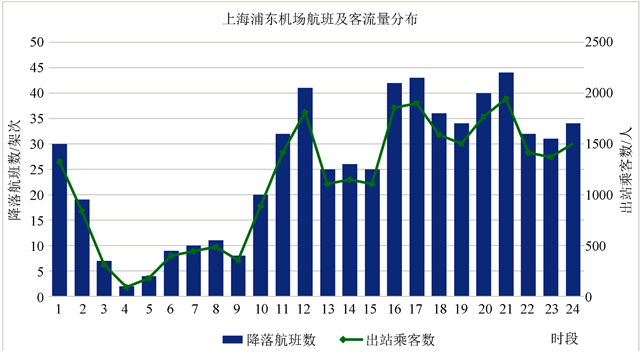Figure 4. Number of inbound flights and outbound passengers per hour at Shanghai Pudong AirportTable 3. Schedule for passengers to travel to the load zone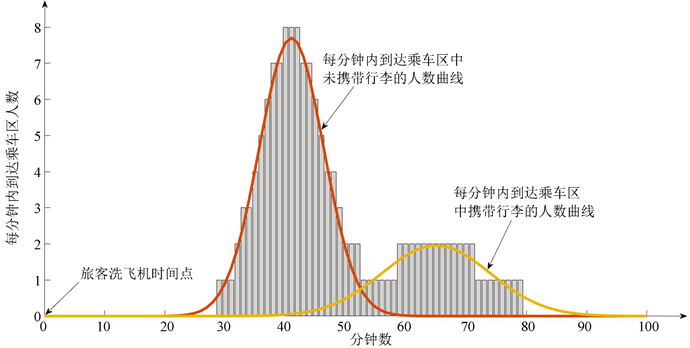Figure 5. Distribution of passenger flow to time in load zone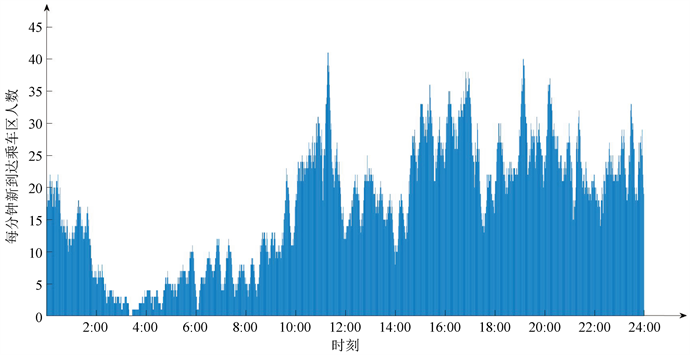Figure 6. Passenger flow per minute in taxi area

2) 最大等待时间Tmax

$\left\{\begin{array}{l}选择行动{a}_{1}的期望收益为E\left({w}_{1}\right)={r}_{i}\left({s}_{1}+{s}_{2}\right)\\ 选择行动{a}_{2}的期望收益为E\left({w}_{2}\right)=\left[{r}_{i}{v}_{c}-{v}_{c}p\left({r}_{i}+{r}_{o}\right)\right]{t}_{b1}+\left[{r}_{i}{s}_{2}-{r}_{o}{s}_{1}-p{s}_{2}\left({r}_{i}+{r}_{o}\right)\right]\end{array}$Table 4. Value of related parameters of expected return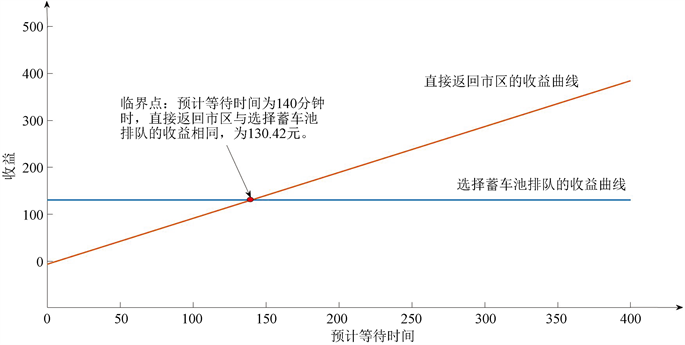Figure 7. Yield curve of different actions

3) 等待时间T

3.3.2. 相关因素的依赖性分析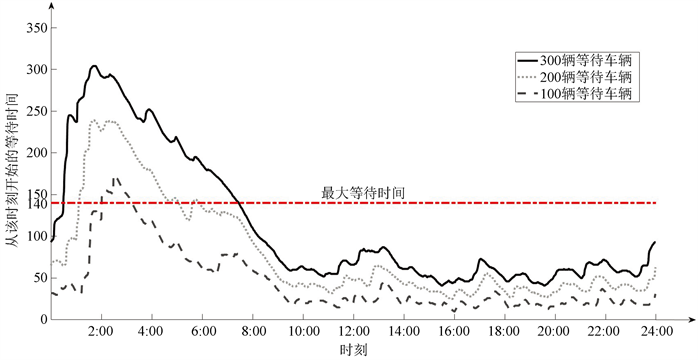Figure 8. Waiting time chart of drivers at different times

4. 优先权分配方案

4.1. 模型的建立

${w}_{a}=\left({r}_{i}-{r}_{o}\right)\underset{i}{\overset{n-1}{\sum }}{x}_{i},n\le 6$(11)

${w}_{b}=-{r}_{o}{x}_{n}+\left[\left(M-\underset{i=1}{\overset{n}{\sum }}{x}_{i}\right)\left(1-p\right){r}_{i}-p{r}_{o}\right]$(12)

$S=\sqrt{\frac{\underset{i=1}{\overset{n}{\sum }}{\left({w}_{i}-\frac{1}{n}\underset{i=1}{\overset{n}{\sum }}{w}_{i}\right)}^{2}}{n-1}}$(13)

$\begin{array}{l}\text{min}S=\sqrt{\frac{\underset{i=1}{\overset{n}{\sum }}{\left({W}_{i}-\frac{1}{n}\underset{i=1}{\overset{n}{\sum }}{W}_{i}\right)}^{2}}{n-1}}\\ \text{s}\text{.t}\text{.}\text{\hspace{0.17em}}\left\{\begin{array}{l}{W}_{i}=W\left({x}_{i},l\right)\\ 8\le l\le 38,l\in N\\ X~N\left(47,159\right)\end{array}\end{array}$(14)

4.2. 优先权安排方案

1) 某次载客距离小于 $l\left(8 的司机再次返回可以享受优先权；

2) 每名司机连续享有优先权的次数不得超过5次；

3) 享有优先权的司机不需要再次排队。

5. 模型的优点

1) 模型的求解通过计算机仿真模拟进行，推广性好，且计算结果和模型的结论符合客观实际，真实可靠。

2) 本文采用了控制变量的思想，改变出租在蓄车池的排队时长，求得在排队加载客总时间内的平均收益；再求得同等时长内如果不等待到达市区的收益。比较此时两种决策收益大小进行决策，原理直观，最终建成的确定型决策模型可信度高。

3) 问题一中，依照乘客从飞机落地到乘客出航站楼(到达出租上车区)各环节用时，准确地以双峰正态分布的形式预测出了一架航班乘客在飞机落地后，到达出租乘车区乘客数随时间的分布。依据一天的航班班次表将每班次出航站楼人数随时间的分布进行叠加，可得到一天中各时刻内到达乘车区的乘客数。模拟精细。

4) 模型参数求解使用到的数据均为官方数据，来源可信度高。

Study on Driver Decision and Management Optimization of Airport Taxi[J]. 统计学与应用, 2020, 09(01): 7-18. https://doi.org/10.12677/SA.2020.91002

1. 1. 王玉民, 颜基义, 潘建均, 等. 决策系统的研究——决策系统模型的重构[J]. 中国软科学, 2018(7): 138-148.

2. 2. 张春红, 王家宇, 田宇. 基于数学期望的决策模型[J]. 职业技术, 2014(9): 94-95.

3. 3. 王志清. 2017年全国民航航班运行效率报告[R]. 北京: 民航局运行监控中心, 2018: 5.

4. 4. 民航出行数据研究中心. 2018年中国民航散客出行报告[EB/OL]. http://www.199it.com/archives/813672.html, 2018-12-26/2019-09-15.

5. 5. 徐鑫, 郭民之, 石峰利. 双峰数据分布的模拟[J]. 云南师范大学学报(自然科学版), 2013, 33(2): 46-51.

6. 6. 张泉峰. 首都机场接续运输协调保障技术研究与实现[D]: [博士学位论文]. 四川: 电子科技大学, 2015.

7. 7. 上海机场有限公司. 上海市出租车收费标准[EB/OL]. https://www.shanghaiairport.com/cn/jcjt/index_53191.html, 2018-09-04/2019-09-15.

8. 8. 上海机场有限公司. 浦东机场出租车里程及参考价格[EB/OL]. https://www.shanghaiairport.com/cn/jcjt/index_53191.html, 2018-09-04/2019-09-15.

9. 9. 王燕, 罗秀秀. 公共蓄车场服务质量测评——以上海浦东机场出租车蓄车场为例[J]. 经济管理, 2013, 35(8): 171-180.

10. 10. 王行宾. 切实解决机场出租车乱象，缓解乘车难[J]. 协商论坛, 2013(8): 30-31.